# 2D NMR simulation (Crystalline solids)¶

The following examples are the NMR spectrum simulation for crystalline solids. The examples include the illustrations for the following methods: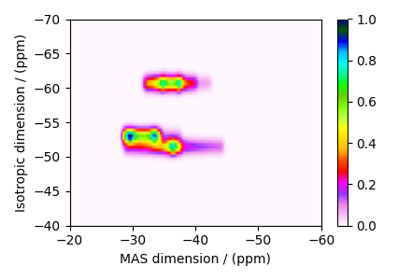RbNO₃, ⁸⁷Rb (I=3/2) 3QMAS

RbNO₃, ⁸⁷Rb (I=3/2) 3QMAS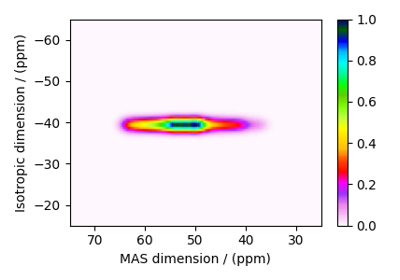Albite, ²⁷Al (I=5/2) 3QMAS

Albite, ²⁷Al (I=5/2) 3QMAS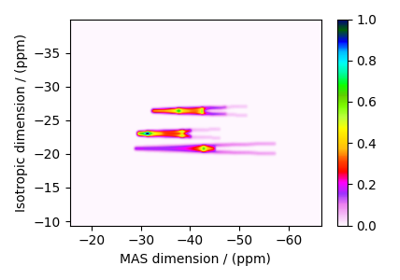RbNO₃, ⁸⁷Rb (I=3/2) STMAS

RbNO₃, ⁸⁷Rb (I=3/2) STMAS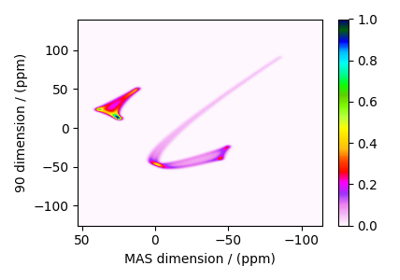Rb₂SO₄, ⁸⁷Rb (I=3/2) SAS

Rb₂SO₄, ⁸⁷Rb (I=3/2) SAS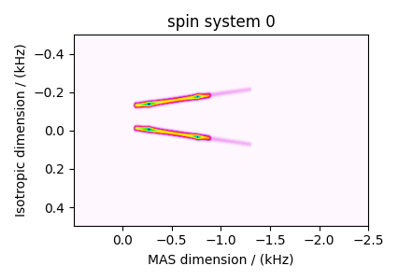Co59 (I=7/2) STMAS

Co59 (I=7/2) STMAS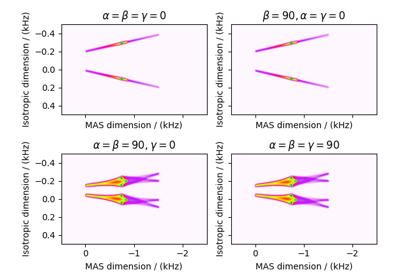Co59 (I=7/2) STMAS

Co59 (I=7/2) STMAS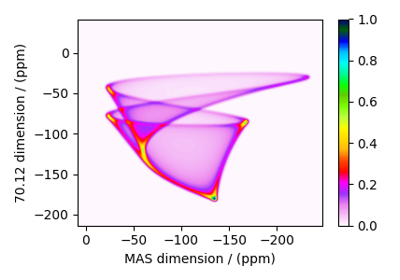Rb₂CrO₄, ⁸⁷Rb (I=3/2) SAS

Rb₂CrO₄, ⁸⁷Rb (I=3/2) SAS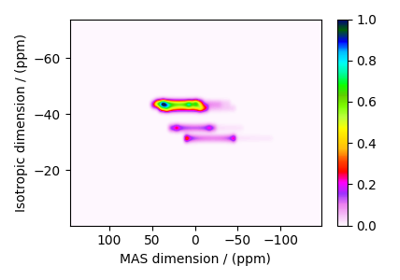Coesite, ¹⁷O (I=5/2) 3QMAS

Coesite, ¹⁷O (I=5/2) 3QMAS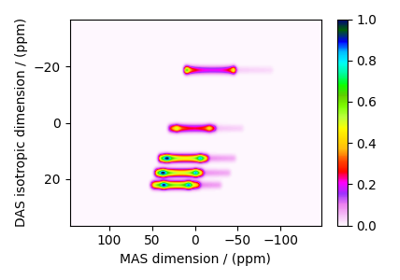Coesite, ¹⁷O (I=5/2) DAS

Coesite, ¹⁷O (I=5/2) DAS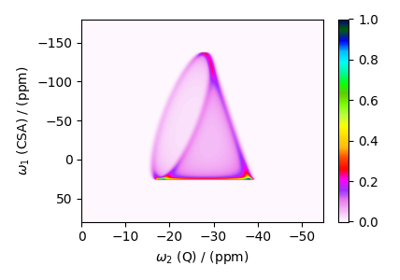Rb₂CrO₄, ⁸⁷Rb (I=3/2) COASTER

Rb₂CrO₄, ⁸⁷Rb (I=3/2) COASTER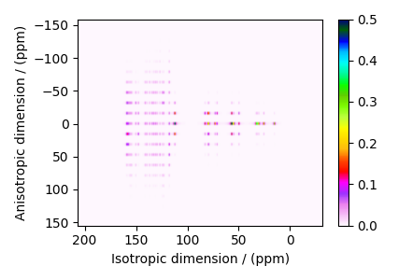Itraconazole, ¹³C (I=1/2) PASS

Itraconazole, ¹³C (I=1/2) PASS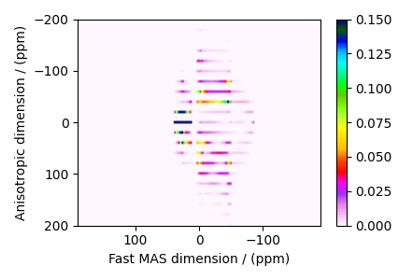Rb₂SO₄, ⁸⁷Rb (I=3/2) QMAT

Rb₂SO₄, ⁸⁷Rb (I=3/2) QMAT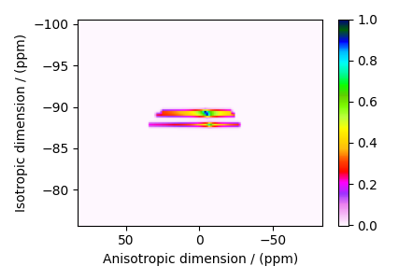Wollastonite, ²⁹Si (I=1/2), MAF

Wollastonite, ²⁹Si (I=1/2), MAF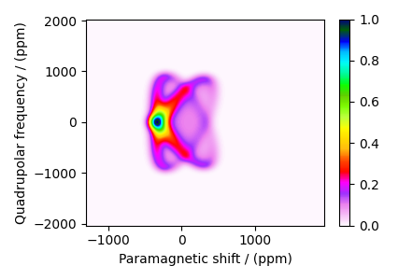MCl₂.2D₂O, ²H (I=1) Shifting-d echo

MCl₂.2D₂O, ²H (I=1) Shifting-d echo